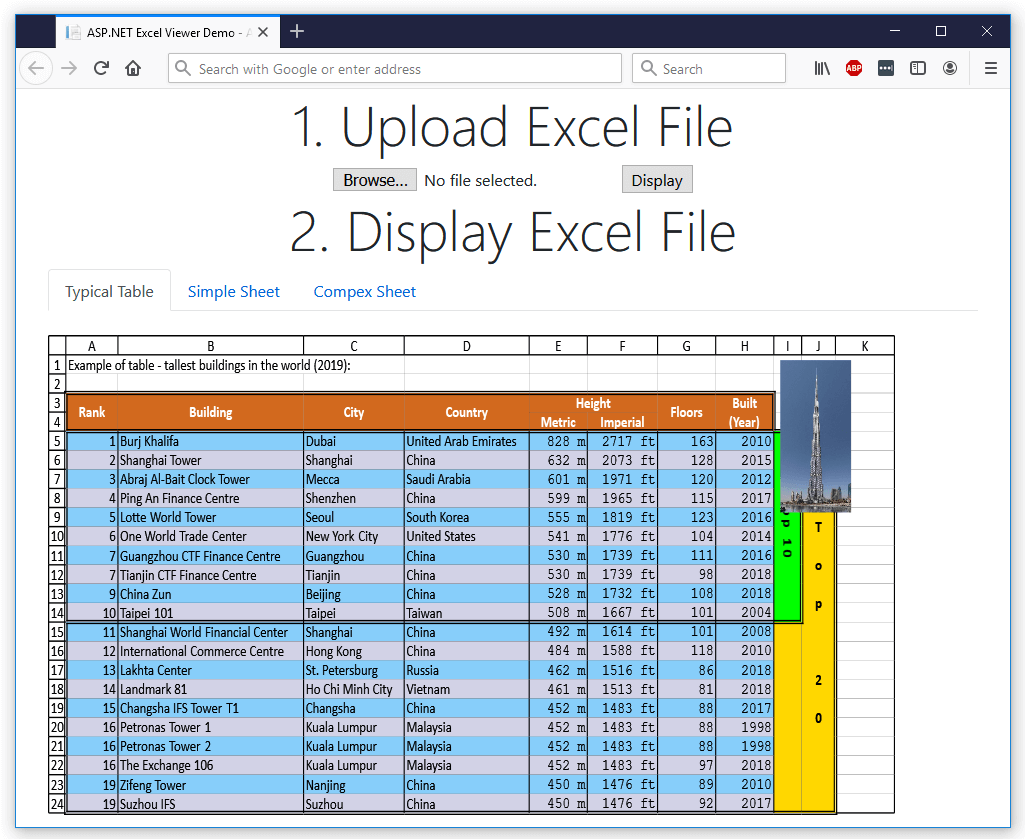# ASP.NET Excel Viewer

If you want to view your Excel files in ASP.NET, you can do this with GemBox.Spreadsheet in two different ways:

• Export each sheet as an image.
• Export the Excel file as an html file.

## Display an Excel file as collection of images

You can display your Excel file by using tabs, where each tab shows an image of the sheet. Here's one way to do this:

• Configure GemBox.Spreadsheet with the option to save to an image as shown in the ASP.NET Core example.
• When a user uploads their file, load it with GemBox.Spreadsheet.
• Save each sheet to an image and store the image on a server.
• On the HTML page, show tab control where the header for the tab is the sheet name.
• The content of each tab should be an `img` element.
• When the page requests an image, pull it from the server.Screenshot of HTML page that displays an Excel file

The code below shows how to do this. It uses an ASP.NET `Session` object for storing images but you can also use a database.

``````@model List<SheetModel>
@{
ViewData["Title"] = "ASP.NET Excel Viewer Demo";
}

<div class="text-center">
<h1 class="display-4">1. Upload Excel File</h1>
@using (Html.BeginForm("UploadFile", "Home", FormMethod.Post, new { enctype = "multipart/form-data" }))
{
<input type="file" name="file" accept=".xlsx,.xls,.ods,.csv,.txt,.xlsm,.xltx,.xltm,.html,.mhtml" />
<input type="submit" value="Display" />
}
<h1 class="display-4">2. Display Excel File</h1>
@if (Model.Count > 0)
{
<ul class="nav nav-tabs" id="myTab" role="tablist">
@for (int i = 0; i < Model.Count; i++)
{
<li class="nav-item">
<a @Html.Raw(i == 0 ? "class='nav-link active'" : "class='nav-link'") id="@("tab"+i)" data-toggle="tab" href="@("#tabpanel"+i)" role="tab" aria-controls="@("tabpanel" + i)" aria-selected="@(i==0)">
@Model[i].SheetName
</a>
</li>
}
</ul>
<div class="tab-content" id="myTabContent">
@for (int i = 0; i < Model.Count; i++)
{
<div @Html.Raw(i == 0 ? "class='tab-pane fade show active'" : "class='tab-pane fade'") id="@("tabpanel" + i)" role="tabpanel" aria-labelledby="@("tab"+i)">
@if (Model[i].HasContent)
{
<img src="@Url.Action("ShowImage", "Home", new { index = i })" class="float-left mt-4" />
}
</div>
}
</div>
}
else
{
}
</div>
``````
``````using AspNetExcelViewer.Models;
using Microsoft.AspNetCore.Http;
using Microsoft.AspNetCore.Mvc;
using Microsoft.Extensions.Logging;
using System;
using System.Collections.Generic;
using System.Diagnostics;
using System.IO;

public class HomeController : Controller
{
private readonly ILogger<HomeController> _logger;

static HomeController()
{
// If you are using the Professional version, enter your serial key below.
}

public HomeController(ILogger<HomeController> logger)
{
_logger = logger;
}

public IActionResult Index()
{
List<SheetModel> sheets = new List<SheetModel>();
int? sheetCount = HttpContext.Session.GetInt32("ExcelFileSheetCount");
if (sheetCount.HasValue)
{
for (int i = 0; i < sheetCount.Value; i++)
{
HttpContext.Session.GetString("ExcelFileSheetName" + i),
HttpContext.Session.Get("ExcelFileSheetImage" + i) != null));
}
}

return View(sheets);
}

// Read stored image from the Session
public ActionResult ShowImage(int index)
{
byte[] image = HttpContext.Session.Get("ExcelFileSheetImage" + index);
return new FileStreamResult(new MemoryStream(image), "image/png");
}

[HttpPost]
public ActionResult UploadFile(IFormFile file)
{
// Load excel file that you have uploaded

// Use Session to store file properties required for displaying it on the page
HttpContext.Session.SetInt32("ExcelFileSheetCount", ef.Worksheets.Count);

for (int i = 0; i < ef.Worksheets.Count; i++)
{
ExcelWorksheet sheet = ef.Worksheets[i];

HttpContext.Session.SetString("ExcelFileSheetName" + i, sheet.Name);

// Print options will be used when exporting sheets to images
sheet.PrintOptions.FitWorksheetHeightToPages = 1;
sheet.PrintOptions.FitWorksheetWidthToPages = 1;
sheet.PrintOptions.PrintGridlines = sheet.ViewOptions.ShowGridLines;

// Export active sheet to an image
ef.Worksheets.ActiveWorksheet = sheet;

Tuple<double, double> pageSize = GetSheetSize(sheet);
if (pageSize.Item1 == 0 || pageSize.Item2 == 0)
continue;

using (MemoryStream imageStream = new MemoryStream())
{
ImageSaveOptions options = new ImageSaveOptions()
{
Format = ImageSaveFormat.Png,
Width = pageSize.Item1,
Height = pageSize.Item2,
CropToContent = true
};
ef.Save(imageStream, options);

HttpContext.Session.Set("ExcelFileSheetImage" + i, imageStream.ToArray());
}
}

return RedirectToAction("Index");
}

private static Tuple<double, double> GetSheetSize(ExcelWorksheet sheet)
{
CellRange usedCellRange = sheet.GetUsedCellRange(true);
if (usedCellRange == null)
return Tuple.Create(0d, 0d);

double width = 0, height = 0;
for (int i = 0; i <= usedCellRange.LastColumnIndex; i++)
{
width += sheet.Columns[i].GetWidth(LengthUnit.Pixel);
}

for (int i = 0; i <= usedCellRange.LastRowIndex; i++)
{
height += sheet.Rows[i].GetHeight(LengthUnit.Pixel);
}

return Tuple.Create(width, height);
}
}

public class SheetModel
{
public string SheetName { get; private set; }
public bool HasContent { get; private set; }

public SheetModel(string sheetName, bool hasContent)
{
this.SheetName = sheetName;
this.HasContent = hasContent;
}
}
``````

## Display an Excel file as an HTML file

With GemBox.Spreadsheet you can export your Excel file as HTML or MHTML file and stream it to a user's web browser, or you can export a sheet as an HTML table and include it in your page.

You can find more about exporting to HTML in the HTML Export example.